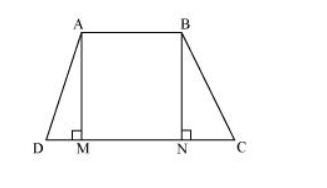# If the non-parallel sides of a trapezium are equal,

Question. If the non-parallel sides of a trapezium are equal, prove that it is cyclic.

Solution:Consider a trapezium $A B C D$ with $A B|| C D$ and $B C=A D$.

Draw AM $\perp C D$ and BN $\perp C D$.

In $\triangle \mathrm{AMD}$ and $\triangle \mathrm{BNC}$,

$A D=B C$ (Given)

$\angle \mathrm{AMD}=\angle \mathrm{BNC}\left(\right.$ By construction, each is $\left.90^{\circ}\right)$

$\mathrm{AM}=\mathrm{BN}$ (Perpendicular distance between two parallel lines is same)

$\therefore \triangle \mathrm{AMD} \cong \triangle \mathrm{BNC}(\mathrm{RHS}$ congruence rule $)$

$\therefore \angle \mathrm{ADC}=\angle \mathrm{BCD}(\mathrm{CPCT}) \ldots(1)$

$\angle B A D$ and $\angle A D C$ are on the same side of transversal $A D$. $\angle B A D+\angle A D C=180^{\circ} \ldots(2)$

$\angle B A D+\angle B C D=180^{\circ}[$ Using equation (1) $]$

This equation shows that the opposite angles are supplementary.

Therefore, ABCD is a cyclic quadrilateral.